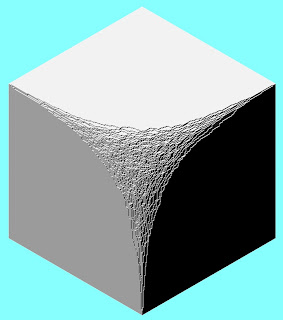## M Theory Lesson 65As nosy snoopy noted, these crystal Calabi-Yau papers are really very interesting. I would like to know more about these random partitions. Okounkov has some notes here.

Recall that in Kapranov’s non-commutative Fourier transform for three coordinates \$x\$, \$y\$ and \$z\$, it is natural to represent monomials by cubical paths traced out on such melting crystal partitions. The two coordinate case goes back to Heisenberg’s original paper, as we have seen. In a modern guise, his sum rule arises in honeycombs, which look a bit like shadows of melting corners.

## 1 Response so far »

1. 1### Doug said,

Hi Kea,
These references may be related to 2003, Andrei Okounkov, ‘The uses of random partitions‘, especially section 5.2.2 Construction of the minimizer.

I think I have referenced this paper before.
Zur Izhakian, ‘Duality of Tropical Curves‘, 31 pages, 4 figures, 2005
Some figures appear to link to honeycombs?
Tropical is usually an alternative name for Min-Plus Algebra, but Max-Plus is discussed.
http://arxiv.org/abs/math/0503691

Zur Izhakian, ‘Tropical Varieties, Ideals and An Algebraic Nullstellensatz’, 27 pages, 2 figures, 2005
http://arxiv.org/abs/math/0511059

Daniele Alessandrini, ‘Amoebas, tropical varieties and compactification of Teichmuller spaces’, 41 pages, 2005
“… every polynomial relation among trace functions on Teichmuller space may be turned automatically in a tropical relation among intersection forms over the boundary.”
http://arxiv.org/abs/math/0505269

Amoeba (mathematics): “In mathematics, an amoeba is a set associated with a polynomial in one or more complex variables. Amoebas have applications in algebraic geometry“ are also discussed in the above references.
http://en.wikipedia.org/wiki/Amoeba_%28mathematics%29

Zur Izhakian,2004, also has ideas about:
Algebraic Curves in Parallel Coordinates – Avoiding the “Over-Plotting” Problem
http://arxiv.org/abs/cs/0403005
and
New Visualization of Surfaces in Parallel Coordinates – Eliminating Ambiguity and Some “Over-Plotting”
http://arxiv.org/abs/cs/0403004